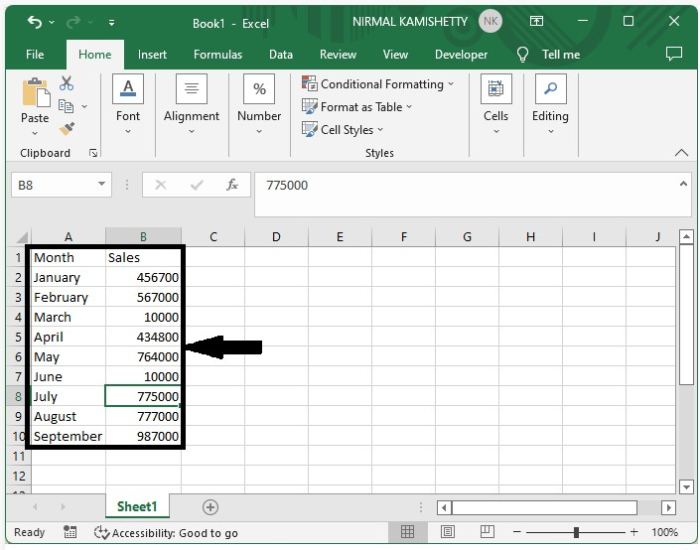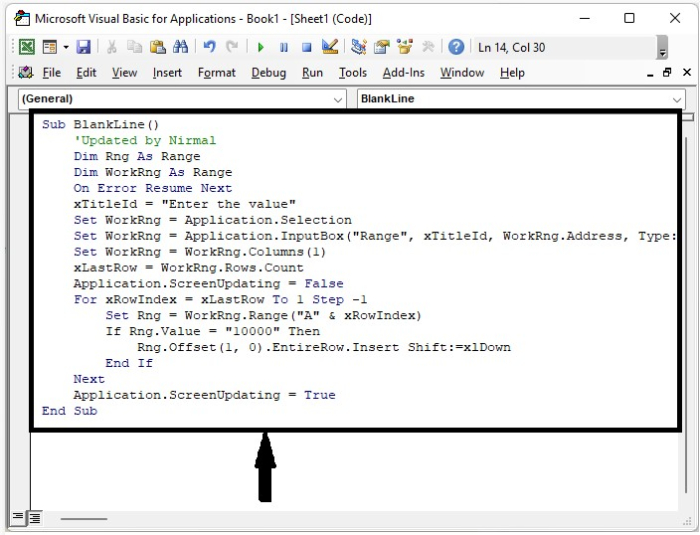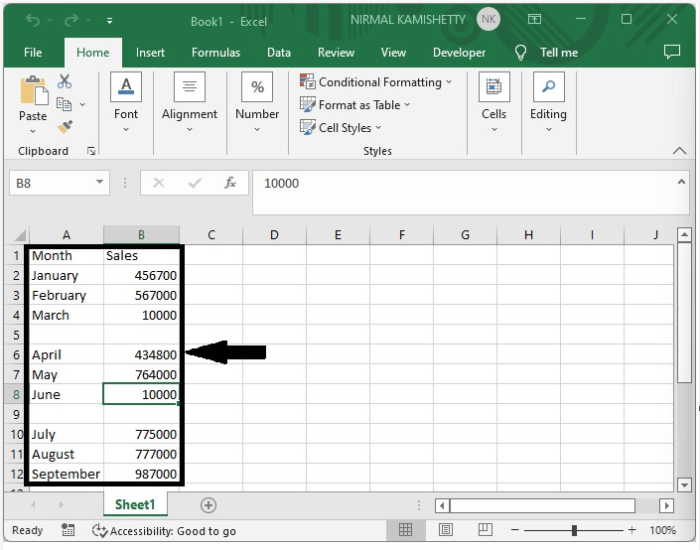# How to Auto-Insert Rows Based on Cell Values in Excel?

Let us assume we have a situation where we want to insert a row below or above a specified value. This process can’t be done by default in Excel, so we will be using the help of the VBA application to complete our process. Read this tutorial to learn how you can autoinsert rows in Excel based on cell values. If we try to complete this task manually, it can be a time-consuming process, as inserting a new row is a multi-step process.

## AutoInsert Rows in Excel Based on Cell Values

Here we will first insert a VBA module and then run the code to complete the task. Let us see a simple process to understand how we can insert a row below based on a cell value in Excel.

Step 1

Let us consider an Excel sheet where the data is similar to the data shown in the below image.Now right-click on the sheet name and select view code to open the vba application, then click on insert and select module, then type the programme into the text box as shown in the below image.

### Example

Sub BlankLine()
'Updated by Nirmal
Dim Rng As Range
Dim WorkRng As Range
On Error Resume Next
xTitleId = "Enter the value"
Set WorkRng = Application.Selection
Set WorkRng = Application.InputBox("Range", xTitleId, WorkRng.Address, Type:=8)
Set WorkRng = WorkRng.Columns(1)
xLastRow = WorkRng.Rows.Count
Application.ScreenUpdating = False
For xRowIndex = xLastRow To 1 Step -1
Set Rng = WorkRng.Range("A" & xRowIndex)
If Rng.Value = "10000" Then
Rng.Offset(1, 0).EntireRow.Insert Shift:=xlDown
End If
Next
Application.ScreenUpdating = True
End Sub


In the code, 10000 is the cell value you want to insert.Step 2

Save the sheet as a macro-enabled worksheet and press the F5 key to run the code. A pop-up window will appear; select the range to which the code should be applied, and our final output will look like the data in the image below.As we can see, the new row is inserted below. To insert the row above the cell, use the following code −

### Example

Sub BlankLine()
'Update by Nirmal
Dim Rng As Range
Dim WorkRng As Range
On Error Resume Next
xTitleId                  = "Enter the range"
Set WorkRng        = Application.Selection
Set WorkRng         = Application.InputBox("Range", xTitleId, WorkRng.Address, Type: = 8)
Set WorkRng         = WorkRng.Columns(1)
xLastRow               = WorkRng.Rows.Count
Application.ScreenUpdating = False
For xRowIndex = xLastRow To 1 Step - 1
Set Rng = WorkRng.Range("A" & xRowIndex)
If Rng.Value = "0" Then
Rng.EntireRow.Insert Shift: = xlDown
End If
Next
Application.ScreenUpdating = True
End Sub


## Conclusion

In this tutorial, we used a simple example to demonstrate how we can insert a row based on cell values in Excel.